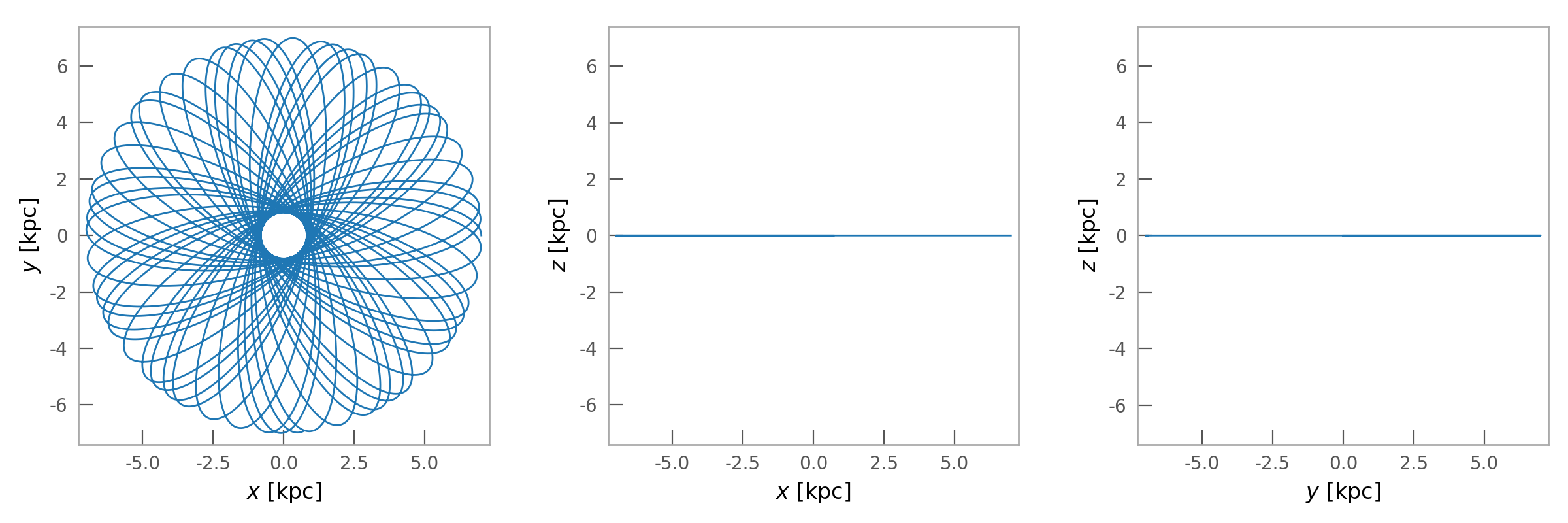# Benefits of Gala¶

>>> import astropy.units as u
>>> import numpy as np
>>> import gala.integrate as gi
>>> import gala.dynamics as gd
>>> import gala.potential as gp
>>> from gala.units import galactic


## Commonly used Galactic gravitational potentials¶

Create potential objects that know how to compute the gradient, energy, etc. at a given position:

>>> pot = gp.IsochronePotential(m=1E10*u.Msun, b=15.*u.kpc, units=galactic)
>>> pot.energy([8.,6.,7.]*u.kpc)
<Quantity [-0.00131002] kpc2 / Myr2>
>>> pot.gradient([8.,6.,7.]*u.kpc)
<Quantity [[1.57813742e-05],
[1.18360306e-05],
[1.38087024e-05]] kpc / Myr2>


## Extensible and easy to define new potentials¶

New potentials can be easily defined by subclassing the base potential class, PotentialBase. For faster orbit integration and computation, you can also define potentials with functions that evaluate its derived quantities in C by subclassing CPotentialBase. For fast creation of potentials for quick testing, you can also create a potential class directly from an equation that expresses the potential:

>>> SHOPotential = gp.from_equation("1/2*k*x**2", vars="x", pars="k",
...                                 name='HarmonicOscillator')


(note: this requires sympy).

Classes created this way can then be instantiated and used like any other PotentialBase subclass:

>>> pot = SHOPotential(k=1.)
>>> pot.energy([1.])
<Quantity [0.5]>


## Extremely fast orbit integration¶

Most of the guts of the built-in potential classes are implemented in C, enabling extremely fast orbit integration for single or composite potentials:

>>> pot = gp.IsochronePotential(m=1E10*u.Msun, b=15.*u.kpc, units=galactic)
>>> w0 = gd.PhaseSpacePosition(pos=[7.,0,0]*u.kpc,
...                            vel=[0.,50.,0]*u.km/u.s)
>>> import timeit
>>> timeit.timeit(lambda: gp.Hamiltonian(pot).integrate_orbit(w0, dt=0.5, n_steps=10000), number=100) / 100.
0.0028513244865462184


For a composite potential:

>>> bulge = gp.IsochronePotential(m=2E10*u.Msun, b=0.5*u.kpc, units=galactic)
>>> disk = gp.MiyamotoNagaiPotential(m=6E10*u.Msun, a=3*u.kpc, b=0.26*u.kpc, units=galactic)
>>> pot = gp.CCompositePotential(bulge=bulge, disk=disk)
>>> timeit.timeit(lambda: gp.Hamiltonian(pot).integrate_orbit(w0, dt=0.5, n_steps=10000), number=100) / 100.
0.0031369362445548177


## Precise integrators¶

The default orbit integration routine uses LeapfrogIntegrator, but the high-order Dormand-Prince 853 integration scheme is also implemented as DOPRI853Integrator:

>>> orbit = gp.Hamiltonian(pot).integrate_orbit(w0, dt=0.5, n_steps=10000,
...                             Integrator=gi.DOPRI853Integrator)


## Easy visualization¶

Numerically integrated orbits can be easily visualized using the plot() method:

>>> orbit.plot()## Astropy units support¶

All functions and classes have Astropy unit support built in: they accept and return Quantity objects wherever possible. In addition, this package uses an experimental new UnitSystem class for storing systems of units and default representations.